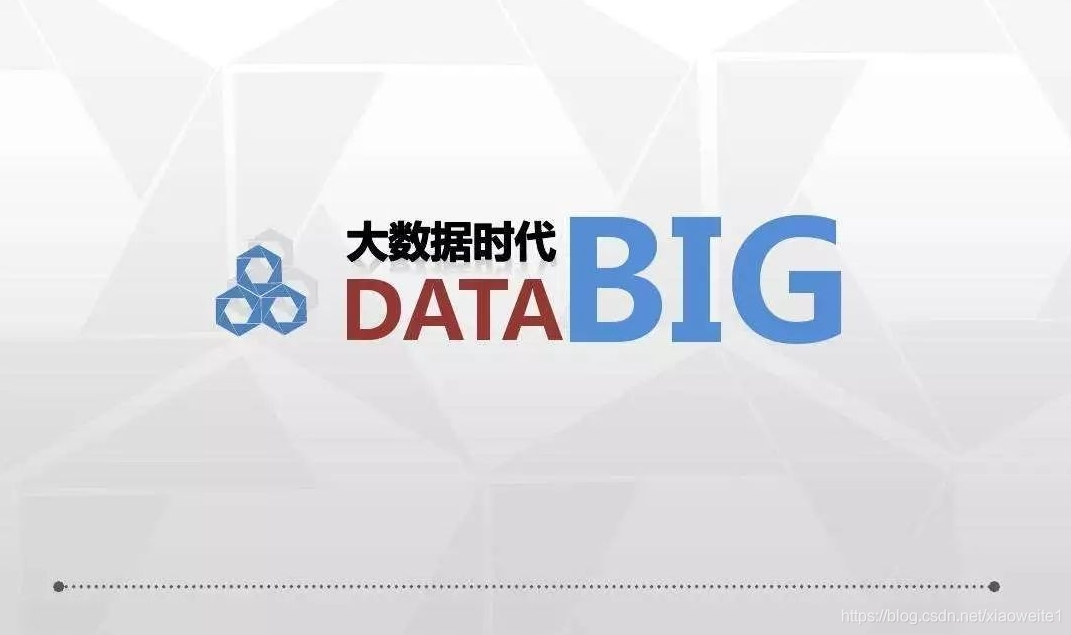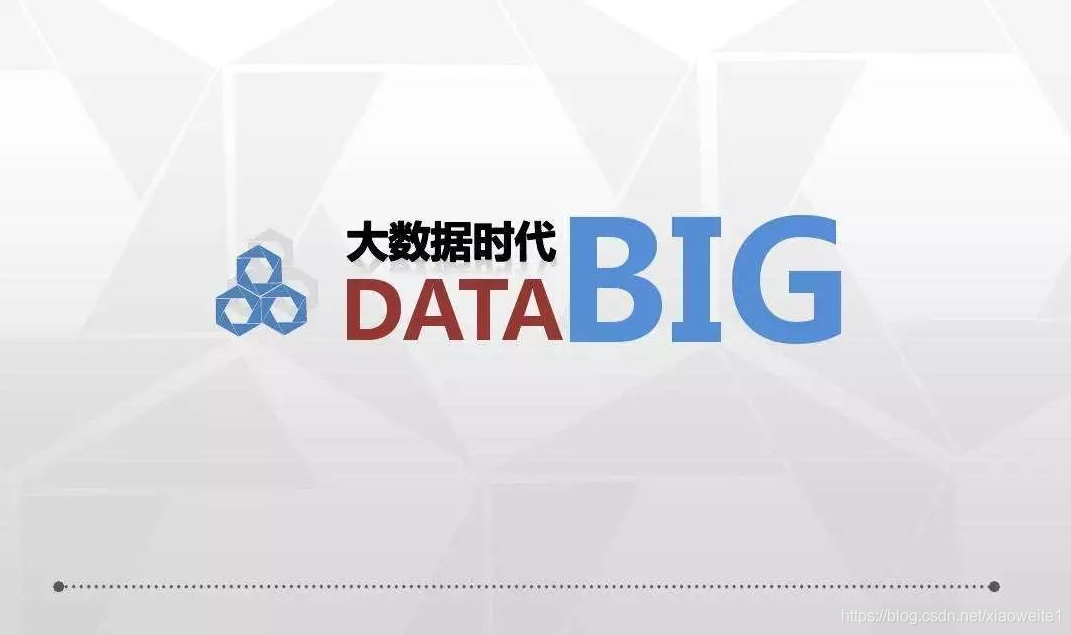# 大数据基础（一）：大数据概念

• 2022 年 7 月 12 日
• 本文字数：951 字

阅读完需：约 3 分钟# ​大数据概念## 常见数据存储单位

1Byte = 8bit    1K(千)= 1024Byte  1MB（兆)=1024K  1G(吉) = 1024M

1T(太)=1024G   1P(拍)=1024T     1E(艾)=1024P     1Z(泽)=1024E

1Y (尧)=1024Z   1B(布)=1024Y     1N(诺)=1024B    1D(刀)=1024N

## 大数据## 评论